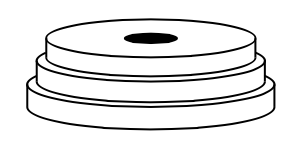### Home > APCALC > Chapter 8 > Lesson 8.1.2 > Problem8-24

8-24.While working out, Warren wants to know the volume of the ten weights he is lifting. Each weight is a circular disk roughly $2$ inches thick. Also, there is a circular hole (diameter $1$ inch) at the center of each weight for the barbell. If the weights were stacked from largest to smallest, the radius of each disk is $\frac { 1 } { 2 }$-inch smaller than the next larger size. (Three of the weights are shown at right.) The largest weight has a diameter of $20$ inches. The next weight has a diameter $19$ inches, etc.

1. What is the radius of the largest disk?

The given information involves diameters, not radii.

2. What is the radius of the hole in the center?

Refer to the hint in part (a).

3. What is the formula for the volume of the largest disk, in words?

Each disk is a cylinder with a hole in the middle. How would you compute the volume of such a figure?

4. Write an expression for the volume of each disk if $i$ represents the number of times the radius is reduced?

$V=\pi \left ( 10-\frac{i}{2} \right )^{2}(2)-\pi \left ( \frac{1}{2} \right )^{2}(2)$

5. Use summation notation to write an expression that will calculate the volume of the weights Warren is lifting.

Recall that the index will account for each and every weight. How many weights are there?

6. Calculate the total volume.